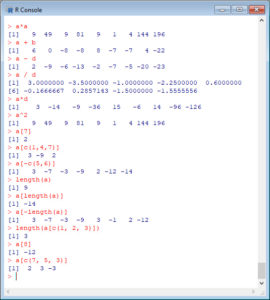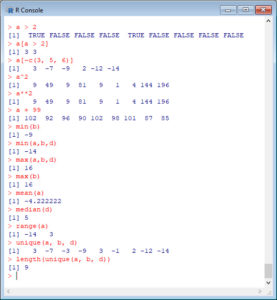In Part 8, let’s look at some basic commands in R.

Set up the following vectors by cutting and pasting from this document:

```a <- c(3,-7,-3,-9,3,-1,2,-12, -14) b <- c(3,7,-5, 1, 5,-6,-9,16, -8) d <- c(1,2,3,4,5,6,7,8,9)```

Now figure out what each of the following commands do. You should not need me to explain each command, but I will explain a few.

`a*a`

``` a + b a - d a / d a*d a^2 a a[c(1, 4, 7)] a[-c(5,6)] length(a) a[length(a)] a[-length(a)] length(a[c(1, 2, 3)] a ```

`a[c(7, 5, 3)]````a > 2 a[a > 2] a[-c(3, 5, 6)] a^2 a**2 a + 99 min(b) min(a,b,d) max(a,b,d) max(b) mean(a) median(d) range(a) unique(a, b, d) length(unique(a, b, d))```The syntax `a > 2` gives a logical vector of elements that are either `TRUE` or `FALSE` depending on whether each element meets the criterion of being greater than 2. Then the syntax `a[a > 2]` subsets the vector a to a smaller set of elements that are indeed greater than 2.

You can see that elements are identified and indexed using square brackets. Elements are retained if the indices are positive, and excluded if the indices are negative. Note that `a[length(a)]` picks out the last element in the vector, while `a[-length(a)]` removes the last element. Of course, `length(a)` gives the number of elements in the vector `a`.

Suppose that you wished to remove the last element of a permanently, you could do this:

```a <- a[-length(a)] a```The last element (-14) has been removed.

That wasn’t so hard! In blog 9 we will look at further analytic techniques in R.

See you soon!

David

#### Annex: R codes used

```# Create three vectors, i.e. variables.
a <- c(3,-7,-3,-9,3,-1,2,-12,-14)
b <- c(3,7,-5, 1, 5,-6,-9,16,-8)
d <- c(1,2,3,4,5,6,7,8,9) # Multiply vector with itself element by element. a*a # Add two vectors. a + b # Subtract two vectors. a - d # Divide two vectors element by element. a / d # Multiply two vectors element by element. a*d # Square each element of vector a. a^2 # Seventh element of vector a. a # First, fourth and seventh elements of vector a. a[c(1,4,7)] # Remove the fifth and sixth elements from vector a. a[-c(5,6)] # Length of vector a, i.e. number of elements in vector a. length(a) # The last element of vector a. a[length(a)] # Remove the last element from vector a. a[-length(a)] # Length of subset of vector a, i.e. number of elements in the subset of vector a. length(a[c(1, 2, 3)]) # Eighth element of vector a. a # Seventh, fifth and third elements of vector a. a[c(7, 5, 3)] # Create a logical vector of elements (TRUE or FALSE) depending on whether each element meets the criterion of being greater than 2. a > 2

# Create a subset of elements that are greater than 2.
a[a > 2]

# Remove the third, fifth and sixth elements from vector a.
a[-c(3, 5, 6)]

# Square each element of vector a.
a^2

# Square each element of vector a.
a**2

# Add 99 to each element of vector a.
a + 99

# Minimum value of vector b.
min(b)

# Minimum value of vectors a, b, d.
min(a,b,d)

# Maximum value of vectors a, b, d.
max(a,b,d)

# Maximum value of vector b.
max(b)

# Mean value of vector a.
mean(a)

# Median value of vector d.
median(d)

# Interval of values of vector a, i.e. (min, max).
range(a)

# Returns a vector like vectors a, b and d but with duplicate elements removed.
unique(a, b, d)

# Length of the vector created by unique() command.
length(unique(a, b, d))
```